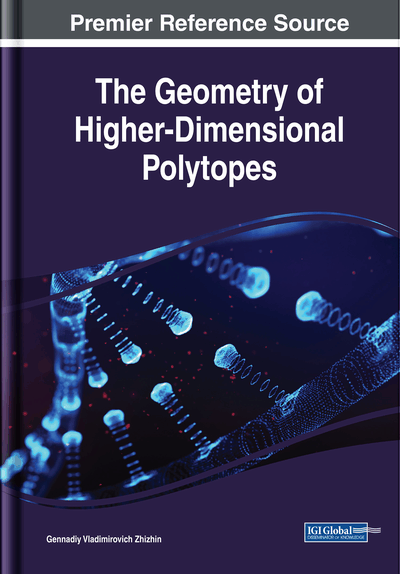# Polytopic Prismahedrons

DOI: 10.4018/978-1-5225-6968-8.ch004
Available
\$37.50
No Current Special Offers

## Abstract

The structure of polytopes - polytopic prismahedrons, which are products of polytopes of lower dimensionality, is investigated. The products of polytopes do not belong to the well-studied class of simplicial polytopes, and therefore their investigations are of independent interest. Analytical dependencies characterizing the structure of the product of polytopes are obtained as a function of the structures of polytope factors. Images of a number of specific polytopic prismahedrons are obtained, tables of structures of polytopic prismahedrons are compiled, depending on the types of polytopes of the factors. The geometric properties of the boundary complexes of polytopic prismehedrons are investigated. Polytopic prismahedrons can be considered as a result of the chemical interaction of molecules, which, from among which there is a polytope of a certain dimension. The possibility of the multivaluedness of the incidence coefficients of geometric elements of polytopic prisms is revealed. It is shown that polytopic prismahedra, due to their nature, as products of polytopes, ensure the filling of n-dimensional spaces during their translation, creating a structure similar to the structure of quasicrystals.
Chapter Preview
Top

## The Structure Of Product Of Polytopes As Function Of Factors Structures

There is the structure of product of polytopes having different structures of their factors is determined.

## Key Terms in this Chapter

Polytopic Prismahedron: The product of polytope by one dimension segment.

6 – Simplex – Polytopic Prismahedron: The product of 4 – simplex by the triangle.

Octahedral Prism: The product of octahedral by one dimension segment.

Tetrahedral Prism: The product of tetrahedral by one dimension segment.

Triangular Prismahedron: The product of triangle by the triangle.

6 – Complex – Tetrahedral Prismahedrons and Octahedral Prismahedrons: The product octahedral by the tetrahedral.

Icosa – Polytopic Prismahedron: The product of icosahedron by the triangle.

Tetrahedral Prismahedron: The product of tetrahedral by the triangle.

Hierarchical and Translation Filling Spaces: At the same time hierarchical and translation filling of a multidimensional spaces.

Dodecahedral Prism: The product of dodecahedral by one dimension segment.

Icosahedral Prism: The product of icosahedral by one dimension segment.

Cube – Polytopic Prismahedron: The product of cube by the triangle.

5 – Cross – Prism: The product of 4 – cross – polytope by one dimension segment.

Octahedral Prismahedron: The product of octahedral by the triangle.

5 – Simplex – Prism: The product of 4 – simplex by one dimension segment.

Dodeca - Polytopic Prismahedron: The product of dodecahedron by the triangle.

N*3 – Angular Prismahedron: The product of n – angle by the triangle.

6 – Complex – Polytopic Prismahedrons: The product of tetrahedron by the tetrahedron.

## Complete Chapter List

Search this Book:
Reset